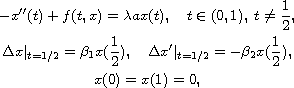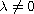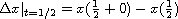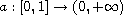Electron. J. Diff. Equ., Vol. 2016 (2016), No. 55, pp. 1-23.

### Global structure of solutions to boundary-value problems of impulsive differential equations Yanmin Niu, Baoqiang Yan

Abstract:
In this article, we study the structure of global solutions to the boundary-value problemwhere,,,, and,are continuous. By a comparison principle and spectral properties of the corresponding linear equations, we prove the existence of solutions by using Rabinowitz-type global bifurcation theorems, and obtain results on the behavior of positive solutions for largewhen.

Submitted January 5, 2016. Published February 25, 2016.
Math Subject Classifications: 34B09, 34B15, 34B37.
Key Words: Comparison arguments; eigenvalues; global bifurcation theorem; multiple solutions; asymptotical behavior of solutions.

Show me the PDF file (294 KB), TEX file, and other files for this article.Yanmin Niu School of Mathematical Sciences Shandong Normal University Jinan 250014, China email: 1398958626@qq.com Baoqiang Yan School of Mathematical Sciences Shandong Normal University Jinan 250014, China email: yanbqcn@aliyun.com